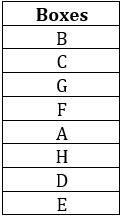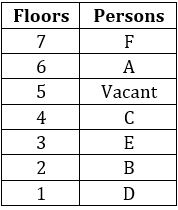Latest Banking jobs   »

# Reasoning Ability Quiz For FCI Phase I 2022- 22nd September

Directions (1-5): Read the following information carefully and answer the following questions.
Eight boxes A, B, C, D, E, F, G and H are place one above the other in any particular order. Box no. 1 is at the bottom and box no. 8 is at the top. Three boxes are placed between A and B. Box H is placed immediately below A. There are two boxes between H and G. There are as many boxes between C and D as between H and B. Box C is kept above D. Box E is kept immediately below box D. Three boxes are there between E and F.

Q1. How many boxes are there above box D?
(a) Four
(b) Three
(c) Six
(d) Two
(e) None of these

Q2. Which of the following box is kept at the top?
(a) B
(b) A
(c) D
(d) E
(e) None of these

Q3. Choose the odd one out?
(a) B-G
(b) G-A
(c) A-D
(d) C-F
(e) E-D

Q4. Which of the following boxes is kept between F and A?
(a) B
(b) G
(c) C
(d) H
(e) None

Q5. How many boxes are there between C and A?
(a) Less than Two
(b) Four
(c) Five
(d) Six
(e) None of these

Directions (6-8): Study the following information carefully and answer the given questions.
Amongst Six friends, A, B, C, D, E and F, each got different marks in the examination. D score marks which are an odd number. D score more marks than E and does not score highest marks. C score more marks than F but less marks than D. C does not score less than E.E score more marks than F and A. A does not score marks that is an odd number. The one who scored the minimum marks scored 31 marks and the one who scored the highest, scored 90 marks.

Q6. Who scored the lowest marks?
(a) F
(b) D
(c) A
(d) B
(e) C

Q7. Who among the following Person has scored third highest marks?
(a) A
(b) C
(c) B
(d) E
(e) Either (a) or (d)

Q8. If C score is 64 then what is possible score of D?
(a) 70
(b) 60
(c) 68
(d) 92
(e) 65

Q9. Rohit ranked 14th from the top and 32nd from the bottom in a class. How many students are there in the class?
(a) 45
(b) 46
(c) 47
(d) 48
(e) None of the above

Q10. Harish ranked 21st from the bottom and 11th from the top among those who passed an exam. Ten boys did not participate in the exam and three failed in it. How many boys were there in the class?
(a) 40
(b) 44
(c) 50
(d) 55
(e) 58

Direction (11-15) Read the information carefully and answer the questions given below:
Six people A, B, C, D, E, and F live on seven different floors of a building (such as ground floor numbered as 1 and top is numbered as 7 and 1 floor is vacant). E lives on an odd numbered floor. D lives on an odd numbered floor below E’s floor. F lives just above A, who doesn’t live on an odd numbered floor. F doesn’t live on 5th floor. C lives on even numbered floor above E’s floor. Numbers of floors between B and C is equal to numbers of floors between E and D.

Q11. Which of the following floor is vacant floor?
(a) second
(b) third
(c) fourth
(d) fifth
(e) None of these

Q12. Who among the following lives on third floor?
(a) C
(b) D
(c) E
(d) F
(e) None of these

Q13. B lives on which of the following floor?
(a) First
(b) Second
(c) Fourth
(d) Third
(e) None of these

Q14. How many persons are live between B and A?
(a) One
(b) Two
(c) Four
(d) Three
(e) None

Q15. Which of the following statement is not true about C?
(a) F lives one of the floors above C’s floor
(b) C lives on an even numbered floor
(c) C lives immediate above vacant floor
(d) Only One floor between C and A
(e) Both (b) and (d)

Solutions

Solutions (1-5):
Sol.S1. Ans. (c)
S2. Ans. (a)
S3. Ans. (e)
S4. Ans. (e)
S5. Ans. (e)

Solutions (6-8):
Sol. B (90)>D>C>E>A>F (31)
S6. Ans. (a)
S7. Ans. (b)
S8. Ans. (e)

S9. Ans. (a)
Sol. Clearly, number of students in the class = (14 + 32-1) = 45.

S10. Ans. (b)
Sol. Number of boys who passed = (21+11-1) = 31.
∴ Total number of boys in the class = (31 + 10 + 3) = 44.

Solutions (11-15):
Sol.S11. Ans. (d)
S12. Ans. (c)
S13. Ans. (b)
S14. Ans. (b)
S15. Ans. (c)#### Congratulations!Download Hindu Review of October 2021: Free PDF